﻿ 含直流潮流控制器的多端高压直流输电网络的小信号稳定性分析及控制系统设计 Small-Signal Stability Analysis and Control System Design of a Meshed Multi-Terminal High-Voltage Direct Current Grid with a Current Flow Controller

Journal of Electrical Engineering
Vol. 07  No. 01 ( 2019 ), Article ID: 29262 , 9 pages
10.12677/JEE.2019.71005

Small-Signal Stability Analysis and Control System Design of a Meshed Multi-Terminal High-Voltage Direct Current Grid with a Current Flow Controller

Xiaolei Sun, Wei Yang

College of Automation, Nanjing University of Science and Technology, Nanjing Jiangsu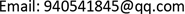Received: Feb. 21st, 2019; accepted: Mar. 7th, 2019; published: Mar. 15th, 2019ABSTRACT

In a high-voltage direct current (HVDC) grid, a DC power flow controller can be used to control the branch current, but it also affects the stability of the entire HVDV system. Therefore, it is necessary to study the dynamic characteristics of HVDC grid with a DC current flow controller (CFC). This paper focuses on the small-signal stability analysis of a three-terminal HVDC system with a DC CFC. Firstly, a small-signal mathematical model is built based on the existing three-terminal HVDC network with a DC CFC, secondly, on this basis, the control strategy for the DC CFC is designed to achieve the stability requirements of the entire system. Finally, a three-terminal HVDC simulation model with a DC CFC is established in Simulink. The proposed control strategy is verified under steady-state and transient operating conditions, and the simulation results are compared with the theoretical values. The dynamic influence of the DC CFC on the HVDC system is also discussed.

Keywords:High-Voltage Direct Current (HVDC), DC Current Flow Controller (CFC), Small-Signal Model, Dynamic Simulation, Stability Analysis1. 引言

2. 含CFC的三端VSC-HVDC系统

2.1. 系统结构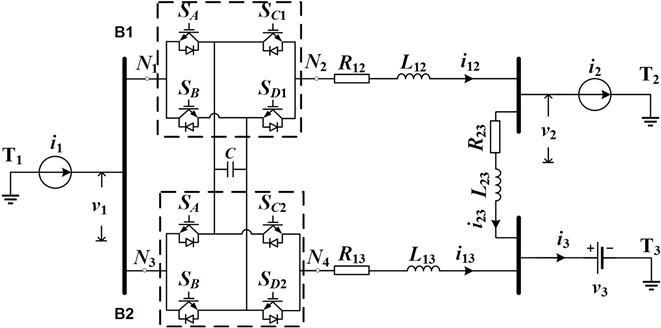Figure 1. Meshed 3-T VSC HVDC grid with the installation of a CFC

2.2. VSC控制策略

VSC能调节换流站端口间的总功率，但并不会改变各直流支路上的电流值。因此，各支路上的电流值会因线路参数的不同而产生不同。在不加以任何控制的情况下，支路电流很容易出现过载现象。为此，就必须在直流线路上加设专门的附加装置来控制各支路的电流，也就是我们所说的直流潮流控制器CFC。

2.3. CFCTable 1. Duty cycles of the PWM signals for the switches in the CFCTable 2. Switch modes of bridge converters in the CFC

3. 系统数学建模

3.1. 系统等效电路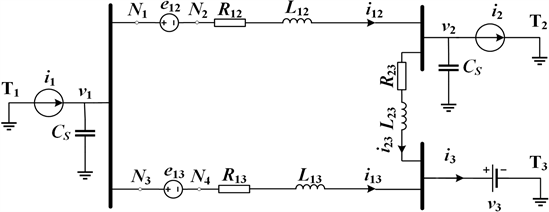Figure 2. Equivalent representation of the whole system

3.2. CFC数学模型

$\begin{array}{l}{e}_{12}=\left({a}_{2}-{a}_{3}\right){u}_{c}\\ {e}_{13}=\left({b}_{2}-{b}_{3}\right){u}_{c}\end{array}$

$C\frac{\text{d}{u}_{c}}{\text{d}t}=\left({a}_{2}-{a}_{3}\right){i}_{12}+\left({b}_{2}-{b}_{3}\right){i}_{13}$

$\begin{array}{l}{e}_{12}=\left({d}_{a}-{d}_{c1}\right){u}_{c}\\ {e}_{13}=\left({d}_{a}-{d}_{c2}\right){u}_{c}\\ C\frac{\text{d}{u}_{c}}{\text{d}t}=\left({d}_{a}-{d}_{c1}\right){i}_{12}+\left({d}_{a}-{d}_{c2}\right){i}_{13}\end{array}$

3.3. HVDC网络数学模型

$\begin{array}{l}{L}_{12}\frac{\text{d}{i}_{12}}{\text{d}t}+{R}_{12}{i}_{12}={v}_{1}-{v}_{2}-{e}_{12}\\ {L}_{13}\frac{\text{d}{i}_{13}}{\text{d}t}+{R}_{13}{i}_{13}={v}_{1}-{v}_{3}-{e}_{13}\\ {L}_{23}\frac{\text{d}{i}_{23}}{\text{d}t}+{R}_{23}{i}_{23}={v}_{2}-{v}_{3}\\ {C}_{s}\frac{\text{d}{v}_{1}}{\text{d}t}={i}_{1}-{i}_{12}-{i}_{13}\\ {C}_{s}\frac{\text{d}{v}_{2}}{\text{d}t}={i}_{12}-{i}_{23}-{i}_{2}\end{array}$

$\begin{array}{l}\stackrel{˙}{x}=Ax+B{v}_{in}\\ y=Cx+D{v}_{in}\end{array}$

$\begin{array}{l}x={\left[\begin{array}{cccccc}{i}_{12}& {i}_{13}& {i}_{23}& {v}_{1}& {v}_{2}& {u}_{c}\end{array}\right]}^{\text{T}}\\ {v}_{in}={\left[\begin{array}{ccc}{i}_{1}& {i}_{2}& {v}_{3}\end{array}\right]}^{\text{T}}\\ y={\left[\begin{array}{cc}{i}_{12}& {u}_{c}\end{array}\right]}^{\text{T}}\end{array}$

4. 小信号分析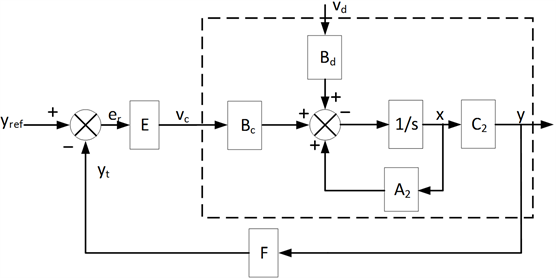Figure 3. System transfer diagram based on the small-signal model

4.1. 开环控制系统

$\begin{array}{l}\stackrel{˙}{x}={A}_{2}x+{B}_{c}{v}_{c}+{B}_{d}{v}_{d}\\ y={C}_{2}x\end{array}$

4.2. 控制环节(E)设计

$E=\left[\begin{array}{cc}\frac{P{I}_{1}}{{i}_{12ref}}& 0\\ 0& \frac{P{I}_{2}}{{i}_{12ref}}\end{array}\right]=\left[\begin{array}{cc}\frac{{K}_{P1}+{K}_{I1}/s}{{i}_{12ref}}& 0\\ 0& -\frac{{K}_{P2}+{K}_{I2}/s}{{u}_{cref}}\end{array}\right]$

4.3. 闭环控制系统

$\begin{array}{l}\stackrel{˙}{x}={A}_{2}x+{B}_{c}E\left({y}_{ref}-F{C}_{2}x\right)+{B}_{d}{v}_{d}\\ y={C}_{2}x\end{array}$

5. 仿真结果Table 3. Parameters of the meshed 3-T VSC-HVDC system

5.1. 工况1：i12ref阶跃变化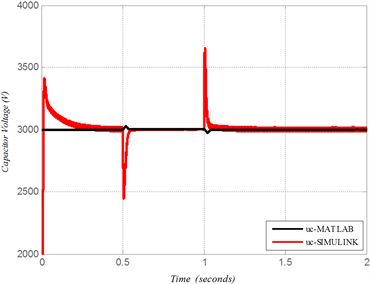Figure 4. System dynamic response to a step change of i12ref

5.2. 工况2：ucref阶跃变化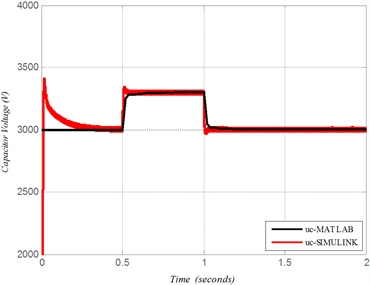Figure 5. System dynamic response to a step change of ucref

6. 结论

1) 本文主要建立了含CFC的三端直流网络的小信号模型，并设计了对CFC的控制环节。基于已建立的小信号模型，此控制环节满足了系统稳定及动态特性的要求。

2) 本文通过与MATLAB/SimPowerSystem仿真结果的比较，验真了小信号模型的准确性；并通过不同工况下的运行结果，验证了本文所设计的控制系统的有效性。

Small-Signal Stability Analysis and Control System Design of a Meshed Multi-Terminal High-Voltage Direct Current Grid with a Current Flow Controller[J]. 电气工程, 2019, 07(01): 44-52. https://doi.org/10.12677/JEE.2019.71005

1. 1. 于亚男, 金阳忻, 江全元, 等. 基于RT-LAB的柔性直流配电网建模与仿真分析[J]. 电力系统保护与控制, 2015, 43(19): 125-130.

2. 2. 孙刚, 时伯年, 赵宇明, 等. 基于MMC的柔性直流配电网故障定位及保护配置研究[J]. 电力系统保护与控制, 2015, 43(22): 127-133.

3. 3. 和敬涵, 李智诚, 王小君. 柔性直流环节对配电网优化运行作用的概率评估[J]. 中国电机工程学报, 2016, 36(2): 342-349.

4. 4. 季一润, 袁志昌, 孙谦浩, 等. 柔性直流配电网典型运行方式及切换方法[J]. 南方电网技术, 2016, 40(13): 141-146.

5. 5. 韩永霞, 何秋萍, 赵宇明, 等. 采用柔性直流技术的智能配电网接入交流电网方式[J]. 电力系统自动化, 2016, 10(4): 8-15.

6. 6. Asplund, G., Lindén, K. and Barker, C. (2013) HVDC Grid Feasibility Study. Electra, 533, 50-59.

7. 7. Vrana, T.K., Yang, Y. and Jovcic, D. (2013) The CIGRE B4 DC Grid Test System. CIGRE Electra Magazine, 270, 10-19.

8. 8. Mu, Q., Liang, J., Lou, Y., et al. (2012) Power Flow Control Devices in DC Grids. IEEE Power and Energy Society General Meeting, San Diego, CA, 22-26 July 2012, 1-7.

9. 9. Jovcic, D., Hajian, M., Zhang, H., et al. (2012) Power Flow Control in DC Transmission Grids Using Mechanical and Semiconductor Based DC/DC Devices. 10th IET International Conference on AC and DC Power Transmission, Birmingham, UK, 19-21 October 2010, 1-6. https://doi.org/10.1049/cp.2012.1972

10. 10. Jovcic, D. and Ooi, B.-T. (2010) Developing DC Transmission Networks Using DC Transformers. IEEE Transactions on Power Delivery, 25, 2535-2543. https://doi.org/10.1109/TPWRD.2010.2052074

11. 11. Kish, G.J., Ranjram, M. and Lehn, P.W. (2015) A Modular Multilevel DC/DC Converter with Fault Blocking Capability for HVDC Interconnects. IEEE Transactions on Power Electronics, 30, 148-162. https://doi.org/10.1109/TPEL.2013.2295967

12. 12. 王朝辉, 王天威, 张军明. 模块化多电平直流变压器研究[J]. 中国电机工程学报, 2016, 36(1): 31-39.

13. 13. 李建国, 赵彪, 宋强, 等. 直流配电网中高频链直流变压器的电压平衡控制策略研究[J]. 中国电机工程学报, 2016, 36(2): 327-334.

14. 14. 孙长江, 张建文, 蔡旭, 等. 隔离型MMC直流变压器的电流源运行[J]. 中国电机工程学报, 2016, 36(7): 1977-1986.

15. 15. Veilleux, E. and Ooi, B.-T. (2012) Multi-Terminal HVDC with Thyristor Power Flow Controller. IEEE Transactions on Power Delivery, 27, 1205-1212. https://doi.org/10.1109/TPWRD.2012.2187463

16. 16. Barker, C.D. and Whitehouse, R.S. (2012) A Current Flow Controller for Use in HVDC Grids. 10th IET International Conference on AC and DC Power Transmission, Birmingham, 1-5.

17. 17. Xu, F. and Xu, Z. (2014) A Modular Multilevel Power Flow Controller for Meshed HVDC Grids. Science China Technological Sciences, 57, 1773-1784. https://doi.org/10.1007/s11431-014-5620-3

18. 18. 姚良忠, 崔红芬, 李官军, 等. 柔性直流电网串联直流潮流控制器及其控制策略研究[J]. 中国电机工程学报, 2016, 36(4): 945-952.

19. 19. Chen, W., Zhu, X., Yao, L., et al. (2015) An Interline DC Power-Flow Controller (IDCPFC) for Multiterminal HVDC System. IEEE Transactions on Power Delivery, 30, 2027-2036. https://doi.org/10.1109/TPWRD.2015.2425412

20. 20. Xu, F. and Xu, Z. (2014) A Modular Multilevel Power Flow Controller for Meshed HVDC Grids. Science China Technological Sciences, 57, 1773-1784. https://doi.org/10.1007/s11431-014-5620-3

21. 21. Chen, W., Zhu, X., Yao, L., Ruan, X., Wang, Z. and Cao, Y. (2015) An Interline DC Power Flow Controller (IDCPFC) for Multiterminal HVDC System. IEEE Transactions on Power Delivery, 30, 2027-2036. https://doi.org/10.1109/TPWRD.2015.2425412

22. 22. Deng, N., Wang, P., Zhang, X.-P., Tang, G.F. and Cao, J.Z. (2015) A DC Current Flow Controller for Meshed Modular Multilevel Converter Multiterminal HVDC Grids. CSEE Journal of Power and Energy Systems, 1, 43-51. https://doi.org/10.17775/CSEEJPES.2015.00006